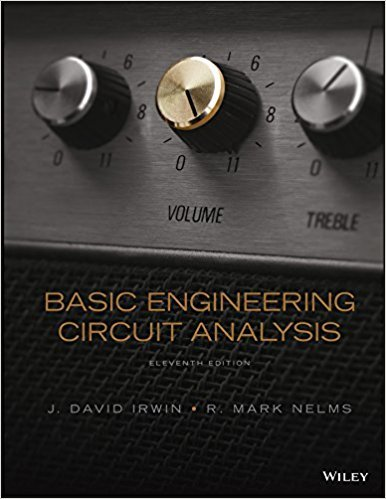×
Get Full Access to Basic Engineering Circuit Analysis - 11 Edition - Chapter 6 - Problem 6.36
Get Full Access to Basic Engineering Circuit Analysis - 11 Edition - Chapter 6 - Problem 6.36

×

# The waveform for the current in the 2-H inductor shown inISBN: 9781118539293 159

## Solution for problem 6.36 Chapter 6

Basic Engineering Circuit Analysis | 11th Edition

• Textbook Solutions
• 2901 Step-by-step solutions solved by professors and subject experts
• Get 24/7 help from StudySoup virtual teaching assistantsBasic Engineering Circuit Analysis | 11th Edition

4 5 1 375 Reviews
28
0
Problem 6.36

The waveform for the current in the 2-H inductor shown in Fig. P6.36a is given in Fig. P6.36b. Determine the following quantities: (a) the energy stored in the inductor at t = 1.5 ms, (b) the energy stored in the inductor at t = 7.5 ms, (c) L(t) at t = 1.5 ms, (d) L(t)at t = 6.25 ms, and (e) L(t) at t = 2.75 ms. 6.34 Draw the waveform for the voltage across a 24-mH inductor when the inductor current is given by the waveform shown in Fig. P6.34. 0.3 t (s) 0.6 1.1 8 4 2 0.9 i(t) (A) Figure P6.34 6.35 The current in a 4-mH inductor is given by the waveform in Fig. P6.35. Plot the voltage across the inductor. 0.5 1.0 0.12 i(t) (mA) t (ms) Figure P6.35 6.31 The current in a 24-mH inductor is given by the waveform in Fig. P6.31. Find the waveform for the voltage across the inductor. 4 5 11 12 12 9 2 12 24 i(t) (A) t (ms) Figure P6.31 6.32 The current in a 10-mH inductor is shown in Fig. P6.32. Determine the waveform for the voltage across the inductor. t (ms) 0 12 1 23456 i(t) (mA) Figure P6.32 6.33 The current in a 50-mH inductor is shown in Fig. P6.33. Find the voltage across the inductor. i(t) (mA) t (ms) 0 20 +10 20 40 60 70 80 Figure P6.33 i(t) 2 H (a) L(t) + t (ms) 7.5 30 10 1 (b) 2 3 4 5 678 i(t) (mA) Figure P6.36

Step-by-Step Solution:
Step 1 of 3

Week 2 Monday, August 28, 202:00 PM Monday, August 28, 2017 2:12 PM Monday, August 28, 2017 2:22 PM Monday, August 28, 2017 2:31 PM Monday, August 28, 2017 2:47 PM Wednesday, August 30, 2017 1:57 PM Wednesday, August 30, 2017 2:13 PM Wednesday, August 30, 2017 2:32 PM Friday, September 1, 2017 2:01 PM Friday, September 1, 2017 2:22 PM Friday, September 1, 2017 2:37 PM

Step 2 of 3

Step 3 of 3

##### ISBN: 9781118539293

Basic Engineering Circuit Analysis was written by and is associated to the ISBN: 9781118539293. The full step-by-step solution to problem: 6.36 from chapter: 6 was answered by , our top Engineering and Tech solution expert on 11/23/17, 05:00AM. This textbook survival guide was created for the textbook: Basic Engineering Circuit Analysis, edition: 11. The answer to “The waveform for the current in the 2-H inductor shown in Fig. P6.36a is given in Fig. P6.36b. Determine the following quantities: (a) the energy stored in the inductor at t = 1.5 ms, (b) the energy stored in the inductor at t = 7.5 ms, (c) L(t) at t = 1.5 ms, (d) L(t)at t = 6.25 ms, and (e) L(t) at t = 2.75 ms. 6.34 Draw the waveform for the voltage across a 24-mH inductor when the inductor current is given by the waveform shown in Fig. P6.34. 0.3 t (s) 0.6 1.1 8 4 2 0.9 i(t) (A) Figure P6.34 6.35 The current in a 4-mH inductor is given by the waveform in Fig. P6.35. Plot the voltage across the inductor. 0.5 1.0 0.12 i(t) (mA) t (ms) Figure P6.35 6.31 The current in a 24-mH inductor is given by the waveform in Fig. P6.31. Find the waveform for the voltage across the inductor. 4 5 11 12 12 9 2 12 24 i(t) (A) t (ms) Figure P6.31 6.32 The current in a 10-mH inductor is shown in Fig. P6.32. Determine the waveform for the voltage across the inductor. t (ms) 0 12 1 23456 i(t) (mA) Figure P6.32 6.33 The current in a 50-mH inductor is shown in Fig. P6.33. Find the voltage across the inductor. i(t) (mA) t (ms) 0 20 +10 20 40 60 70 80 Figure P6.33 i(t) 2 H (a) L(t) + t (ms) 7.5 30 10 1 (b) 2 3 4 5 678 i(t) (mA) Figure P6.36” is broken down into a number of easy to follow steps, and 258 words. Since the solution to 6.36 from 6 chapter was answered, more than 347 students have viewed the full step-by-step answer. This full solution covers the following key subjects: inductor, waveform, fig, current, figure. This expansive textbook survival guide covers 15 chapters, and 1430 solutions.

Unlock Textbook Solution